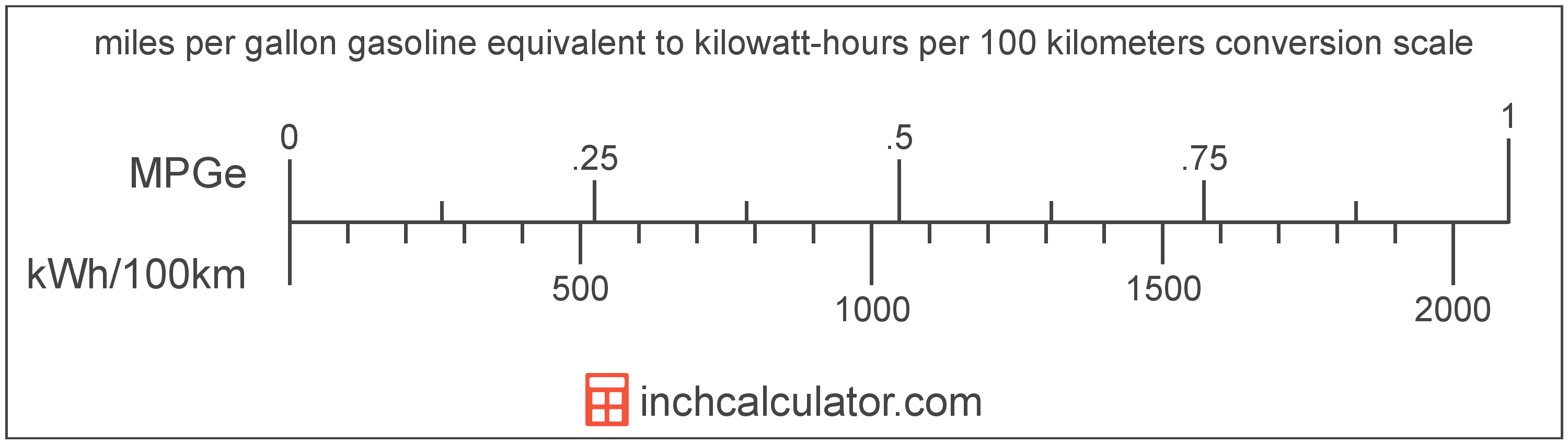# Kilowatt-Hours per 100 Kilometers to Miles per Gallon Gasoline Equivalent Converter (KWh/100km to MPGe)

Enter the electric car efficiency in kilowatt-hours per 100 kilometers below to get the value converted to miles per gallon gasoline equivalent.

Results in Miles per Gallon Gasoline Equivalent:1 kWh/100km = 2,094.331603 MPGe

Do you want to convert MPGe to kWh/100km?

## How to Convert Kilowatt-Hours per 100 Kilometers to Miles per Gallon Gasoline Equivalent

Convert kilowatt-hours per 100 kilometers to miles per gallon gasoline equivalent with this simple formula:

MPGe = 3370.5 ÷ 1.609344 ÷ kWh/100km

Insert the kWh/100km efficiency measurement in the formula and then solve to find the result.

For example, let's convert 50 kWh/100km to MPGe:

50 kWh/100km = ( 3370.5 ÷ 1.609344 ÷ 50 ) = 41.886632 MPGeKilowatt-hours per 100 kilometers and miles per gallon gasoline equivalent are both units used to measure electric car efficiency. Keep reading to learn more about each unit of measure.

## What Are Kilowatt-Hours per 100 Kilometers?

Kilowatt-hours per 100 kilometers are a measure of electric vehicle energy efficiency equal to the kilowatt-hours of energy needed for a vehicle to travel 100 kilometers.

The kilowatt-hour per 100 kilometers is an SI unit of electric car efficiency in the metric system. Kilowatt-hours per 100 kilometers can be abbreviated as kWh/100km; for example, 1 kilowatt-hour per 100 kilometers can be written as 1 kWh/100km.

In the expressions of units, the slash, or solidus (/), is used to express a change in one or more units relative to a change in one or more other units.

## What Are Miles per Gallon Gasoline Equivalent?

Miles per gallon gasoline equivalent is a way of measuring the energy needed equivalent to a gallon of gasoline to travel a distance in miles.

Because electric vehicles do not consume gasoline, the EPA set an equivalent amount of energy in kilowatt-hours that is equal to one gallon of gasoline. According to the EPA, one gallon of gasoline is equal to 33.705 kilowatt-hours.

The mile per gallon gasoline equivalent is a US customary unit of electric car efficiency. Miles per gallon gasoline equivalent can be abbreviated as MPGe; for example, 1 mile per gallon gasoline equivalent can be written as 1 MPGe.

## Kilowatt-Hour per 100 Kilometers to Mile per Gallon Gasoline Equivalent Conversion Table

Table showing various kilowatt-hour per 100 kilometers measurements converted to miles per gallon gasoline equivalent.
Kilowatt-hours Per 100 Kilometers Miles Per Gallon Gasoline Equivalent
0.001 kWh/100km 2,094,332 MPGe
0.002 kWh/100km 1,047,166 MPGe
0.003 kWh/100km 698,111 MPGe
0.004 kWh/100km 523,583 MPGe
0.005 kWh/100km 418,866 MPGe
0.006 kWh/100km 349,055 MPGe
0.007 kWh/100km 299,190 MPGe
0.008 kWh/100km 261,791 MPGe
0.009 kWh/100km 232,704 MPGe
0.01 kWh/100km 209,433 MPGe
0.02 kWh/100km 104,717 MPGe
0.03 kWh/100km 69,811 MPGe
0.04 kWh/100km 52,358 MPGe
0.05 kWh/100km 41,887 MPGe
0.06 kWh/100km 34,906 MPGe
0.07 kWh/100km 29,919 MPGe
0.08 kWh/100km 26,179 MPGe
0.09 kWh/100km 23,270 MPGe
0.1 kWh/100km 20,943 MPGe
0.2 kWh/100km 10,472 MPGe
0.3 kWh/100km 6,981 MPGe
0.4 kWh/100km 5,236 MPGe
0.5 kWh/100km 4,189 MPGe
0.6 kWh/100km 3,491 MPGe
0.7 kWh/100km 2,992 MPGe
0.8 kWh/100km 2,618 MPGe
0.9 kWh/100km 2,327 MPGe
1 kWh/100km 2,094 MPGe

## References

1. United States Environmental Protection Agency, Technology, https://www3.epa.gov/otaq/gvg/learn-more-technology.htm